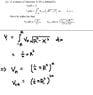# This sequence of functions looks simple but

unscientific

## Homework Statement

The question is attached in the picture.

## The Attempt at a Solution

Since V0 = 1,

Thus V1 = $\stackrel{1}{2}$∏R2 which is a constant.

Then shouldn't Vn be as in the picture?

#### Attachments

•lastqn.jpg
21.9 KB · Views: 383

Sourabh N
I think there is a typo in the question.

$(\sqrt{R^2 - x^2})$ should be $(\sqrt{R^2 - x^2})^n$

unscientific
I think there is a typo in the question.

$(\sqrt{R^2 - x^2})$ should be $(\sqrt{R^2 - x^2})^n$

hmm are u sure about that?

Sourabh N
Yes.

Homework Helper
Dearly Missed

## Homework Statement

The question is attached in the picture.

## The Attempt at a Solution

Since V0 = 1,

Thus V1 = $\stackrel{1}{2}$∏R2 which is a constant.

Then shouldn't Vn be as in the picture?

$$V_1(R) = \int_{-R}^R V_0 \left(\sqrt{R^2-x^2} \right) \, dx = \int_{-R}^R \; 1 \, dx = 2R,$$
etc.

RGV